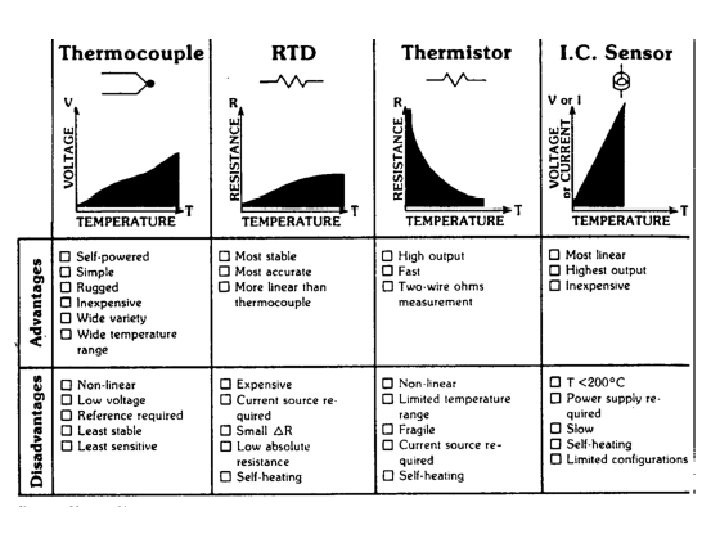Скачать презентацию Temperature Measurement Priyatmadi Temperature Concept Temperature

df9679703381bac507b6fc778d2fef8e.ppt

• Количество слайдов: 48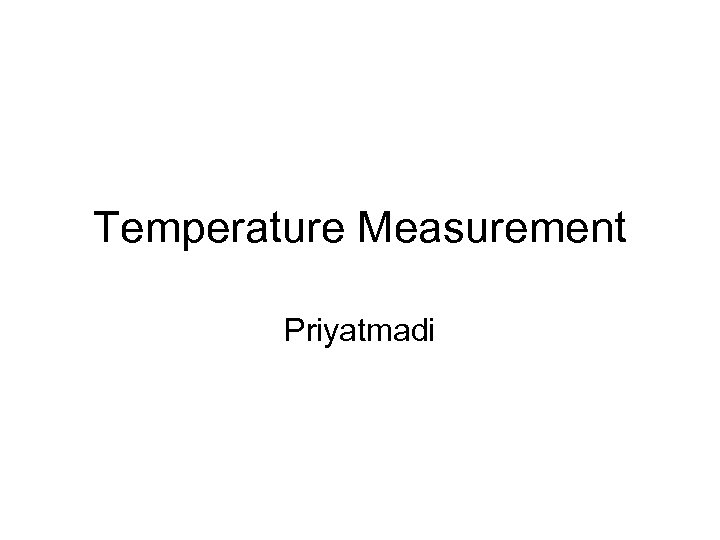Temperature Measurement Priyatmadi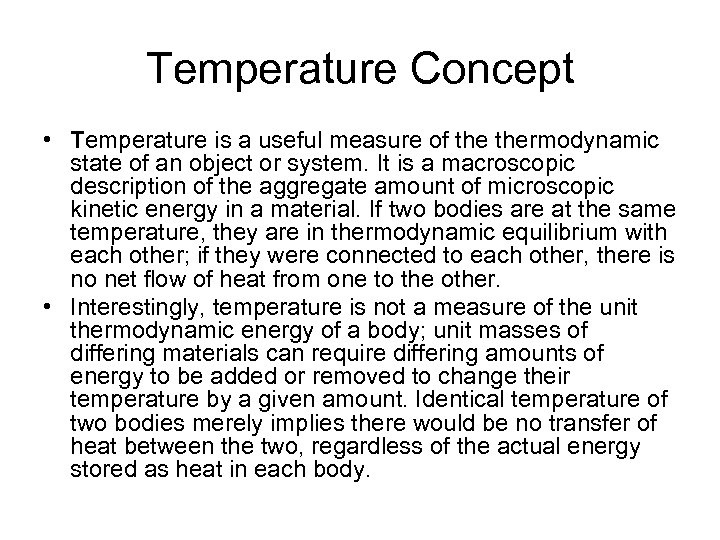Temperature Concept • Temperature is a useful measure of thermodynamic state of an object or system. It is a macroscopic description of the aggregate amount of microscopic kinetic energy in a material. If two bodies are at the same temperature, they are in thermodynamic equilibrium with each other; if they were connected to each other, there is no net flow of heat from one to the other. • Interestingly, temperature is not a measure of the unit thermodynamic energy of a body; unit masses of differing materials can require differing amounts of energy to be added or removed to change their temperature by a given amount. Identical temperature of two bodies merely implies there would be no transfer of heat between the two, regardless of the actual energy stored as heat in each body.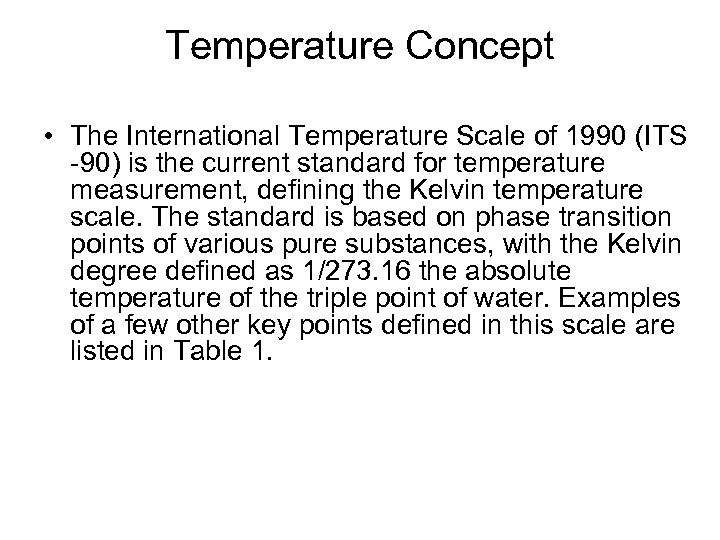Temperature Concept • The International Temperature Scale of 1990 (ITS -90) is the current standard for temperature measurement, defining the Kelvin temperature scale. The standard is based on phase transition points of various pure substances, with the Kelvin degree defined as 1/273. 16 the absolute temperature of the triple point of water. Examples of a few other key points defined in this scale are listed in Table 1.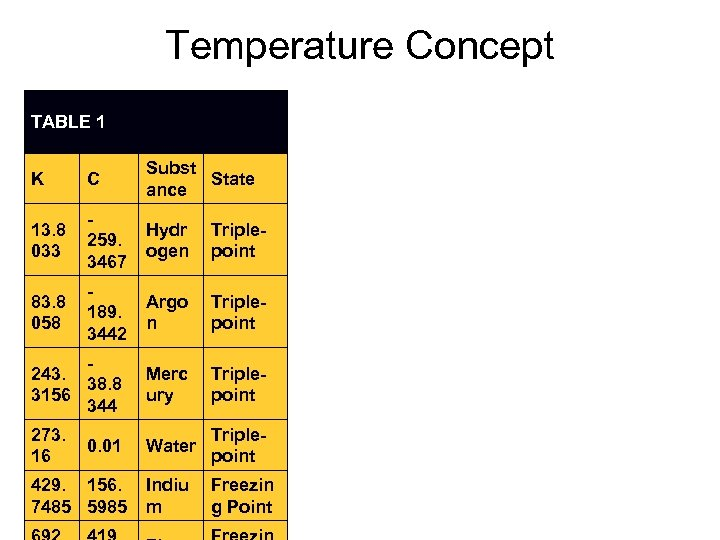Temperature Concept TABLE 1 K C Subst State ance 13. 8 033 259. 3467 Hydr ogen Triplepoint 83. 8 058 189. 3442 Argo n Triplepoint 243. 38. 8 3156 344 Merc ury Triplepoint 273. 16 Water Triplepoint Indiu m Freezin g Point 0. 01 429. 156. 7485 5985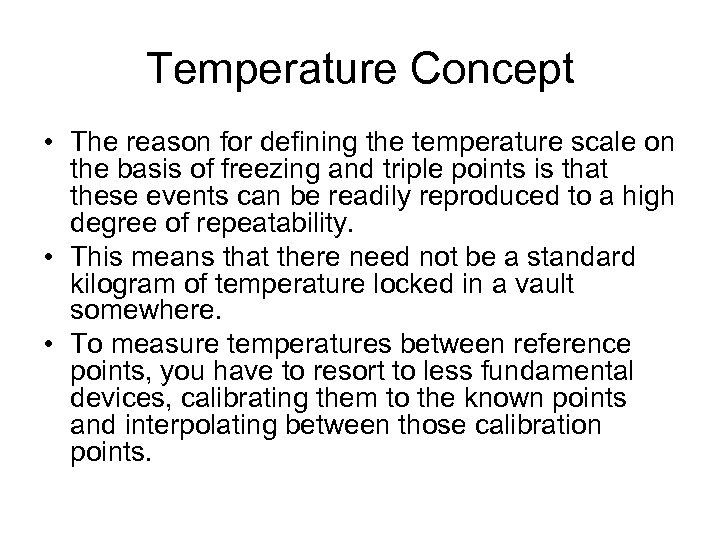Temperature Concept • The reason for defining the temperature scale on the basis of freezing and triple points is that these events can be readily reproduced to a high degree of repeatability. • This means that there need not be a standard kilogram of temperature locked in a vault somewhere. • To measure temperatures between reference points, you have to resort to less fundamental devices, calibrating them to the known points and interpolating between those calibration points.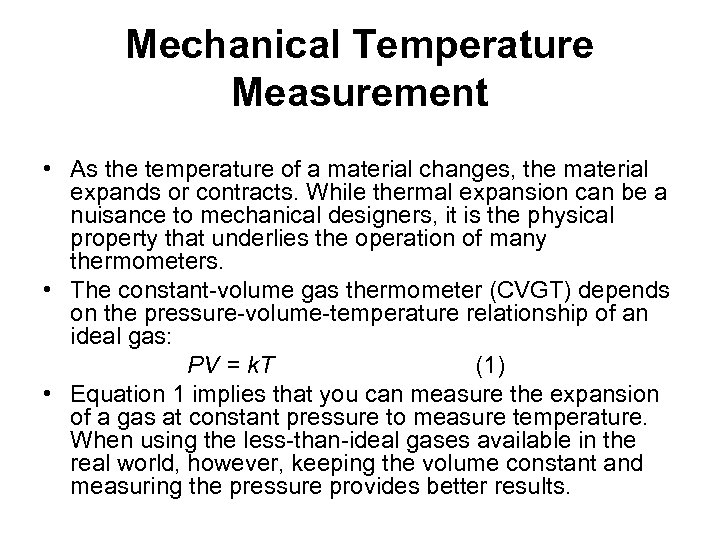Mechanical Temperature Measurement • As the temperature of a material changes, the material expands or contracts. While thermal expansion can be a nuisance to mechanical designers, it is the physical property that underlies the operation of many thermometers. • The constant-volume gas thermometer (CVGT) depends on the pressure-volume-temperature relationship of an ideal gas: PV = k. T (1) • Equation 1 implies that you can measure the expansion of a gas at constant pressure to measure temperature. When using the less-than-ideal gases available in the real world, however, keeping the volume constant and measuring the pressure provides better results.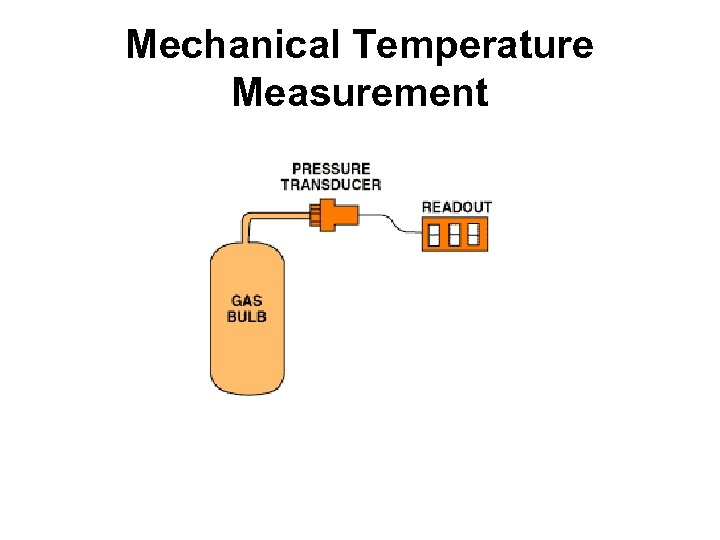Mechanical Temperature Measurement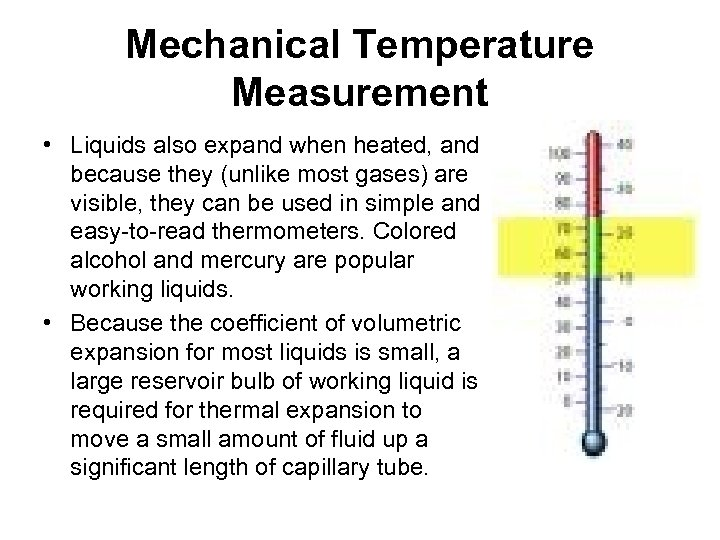Mechanical Temperature Measurement • Liquids also expand when heated, and because they (unlike most gases) are visible, they can be used in simple and easy-to-read thermometers. Colored alcohol and mercury are popular working liquids. • Because the coefficient of volumetric expansion for most liquids is small, a large reservoir bulb of working liquid is required for thermal expansion to move a small amount of fluid up a significant length of capillary tube.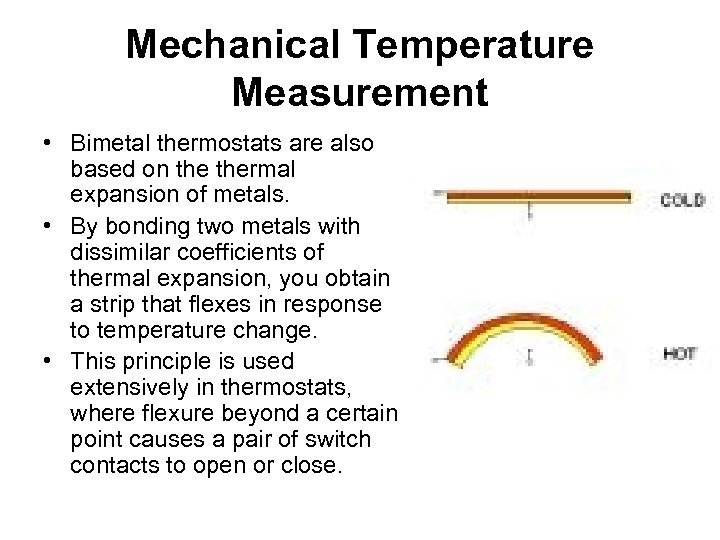Mechanical Temperature Measurement • Bimetal thermostats are also based on thermal expansion of metals. • By bonding two metals with dissimilar coefficients of thermal expansion, you obtain a strip that flexes in response to temperature change. • This principle is used extensively in thermostats, where flexure beyond a certain point causes a pair of switch contacts to open or close.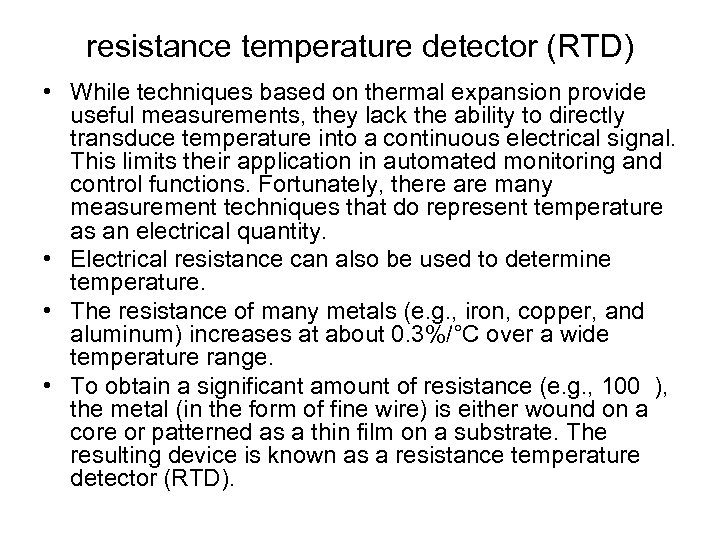resistance temperature detector (RTD) • While techniques based on thermal expansion provide useful measurements, they lack the ability to directly transduce temperature into a continuous electrical signal. This limits their application in automated monitoring and control functions. Fortunately, there are many measurement techniques that do represent temperature as an electrical quantity. • Electrical resistance can also be used to determine temperature. • The resistance of many metals (e. g. , iron, copper, and aluminum) increases at about 0. 3%/°C over a wide temperature range. • To obtain a significant amount of resistance (e. g. , 100 ), the metal (in the form of fine wire) is either wound on a core or patterned as a thin film on a substrate. The resulting device is known as a resistance temperature detector (RTD).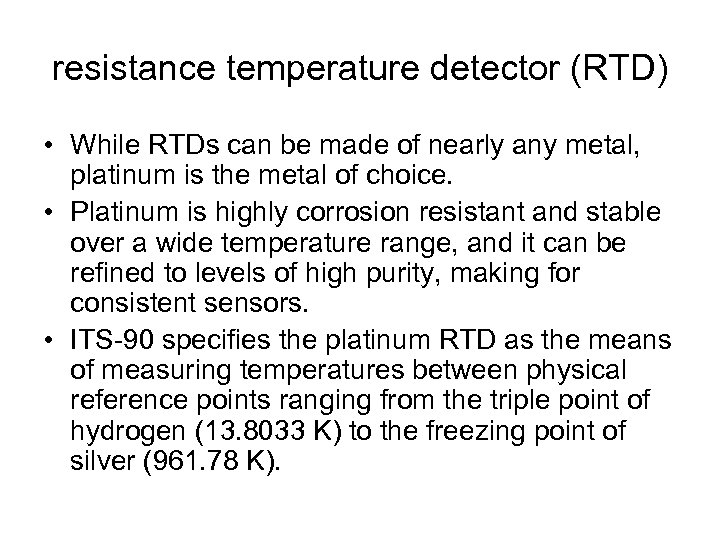resistance temperature detector (RTD) • While RTDs can be made of nearly any metal, platinum is the metal of choice. • Platinum is highly corrosion resistant and stable over a wide temperature range, and it can be refined to levels of high purity, making for consistent sensors. • ITS-90 specifies the platinum RTD as the means of measuring temperatures between physical reference points ranging from the triple point of hydrogen (13. 8033 K) to the freezing point of silver (961. 78 K).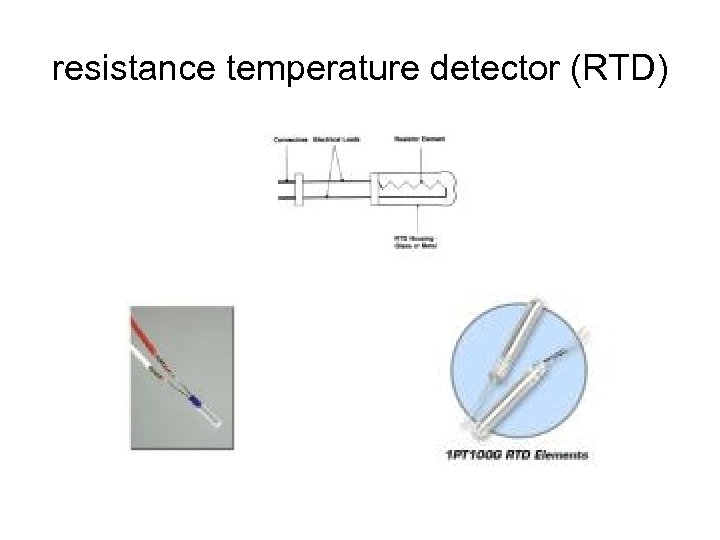resistance temperature detector (RTD)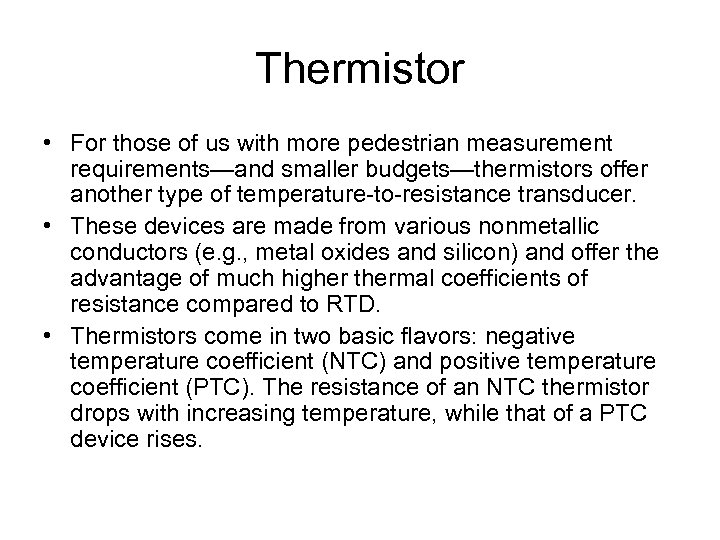Thermistor • For those of us with more pedestrian measurement requirements—and smaller budgets—thermistors offer another type of temperature-to-resistance transducer. • These devices are made from various nonmetallic conductors (e. g. , metal oxides and silicon) and offer the advantage of much higher thermal coefficients of resistance compared to RTD. • Thermistors come in two basic flavors: negative temperature coefficient (NTC) and positive temperature coefficient (PTC). The resistance of an NTC thermistor drops with increasing temperature, while that of a PTC device rises.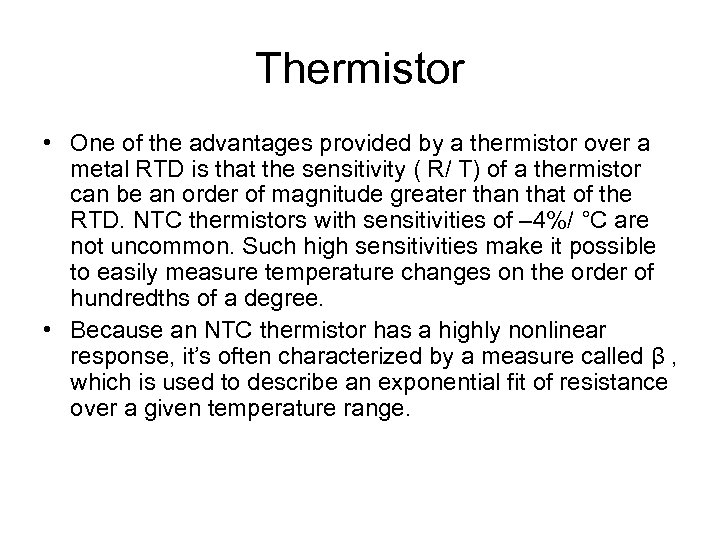Thermistor • One of the advantages provided by a thermistor over a metal RTD is that the sensitivity ( R/ T) of a thermistor can be an order of magnitude greater than that of the RTD. NTC thermistors with sensitivities of – 4%/ °C are not uncommon. Such high sensitivities make it possible to easily measure temperature changes on the order of hundredths of a degree. • Because an NTC thermistor has a highly nonlinear response, it’s often characterized by a measure called β , which is used to describe an exponential fit of resistance over a given temperature range.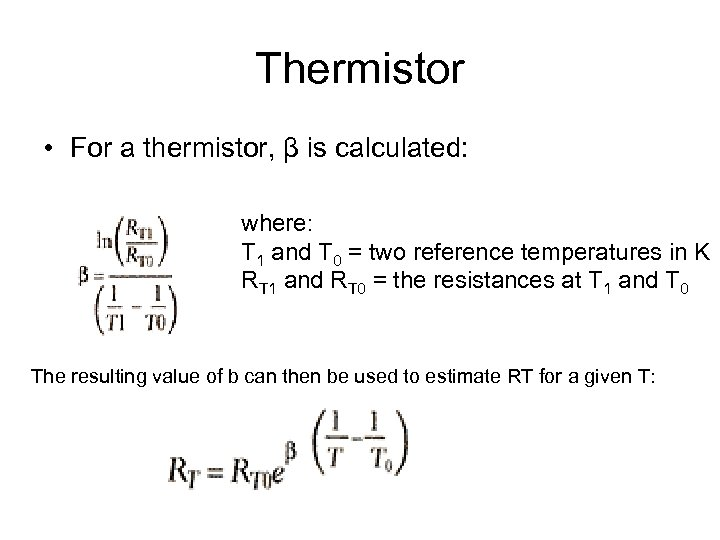Thermistor • For a thermistor, β is calculated: where: T 1 and T 0 = two reference temperatures in K RT 1 and RT 0 = the resistances at T 1 and T 0 The resulting value of b can then be used to estimate RT for a given T: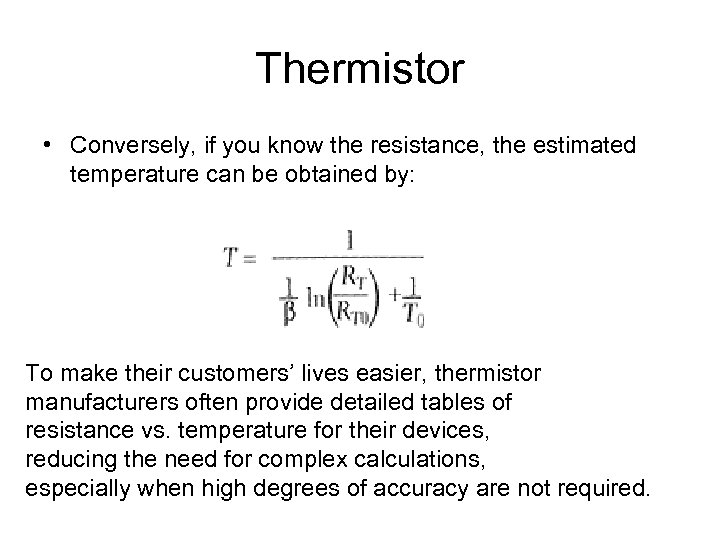Thermistor • Conversely, if you know the resistance, the estimated temperature can be obtained by: To make their customers’ lives easier, thermistor manufacturers often provide detailed tables of resistance vs. temperature for their devices, reducing the need for complex calculations, especially when high degrees of accuracy are not required.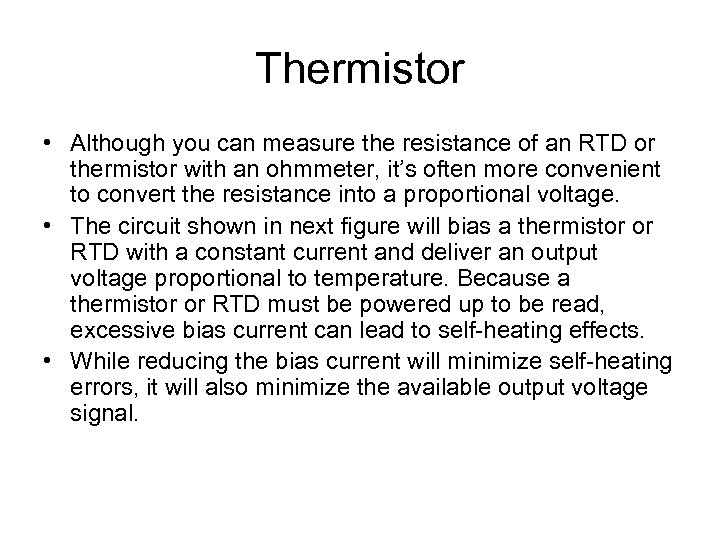Thermistor • Although you can measure the resistance of an RTD or thermistor with an ohmmeter, it’s often more convenient to convert the resistance into a proportional voltage. • The circuit shown in next figure will bias a thermistor or RTD with a constant current and deliver an output voltage proportional to temperature. Because a thermistor or RTD must be powered up to be read, excessive bias current can lead to self-heating effects. • While reducing the bias current will minimize self-heating errors, it will also minimize the available output voltage signal.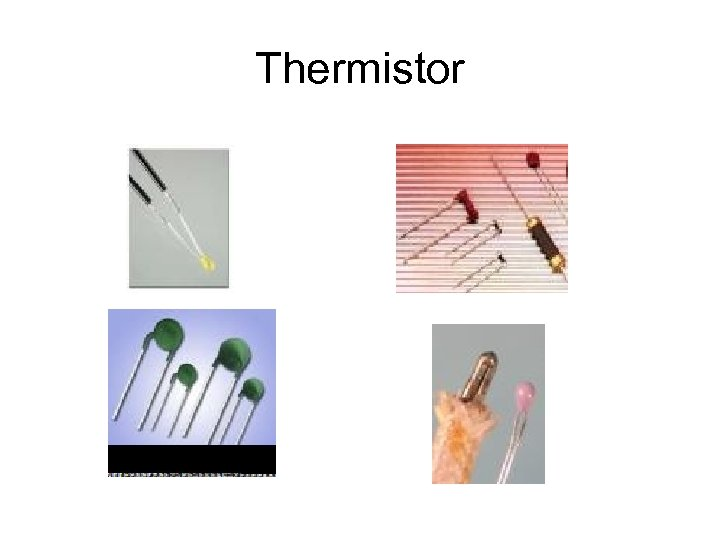Thermistor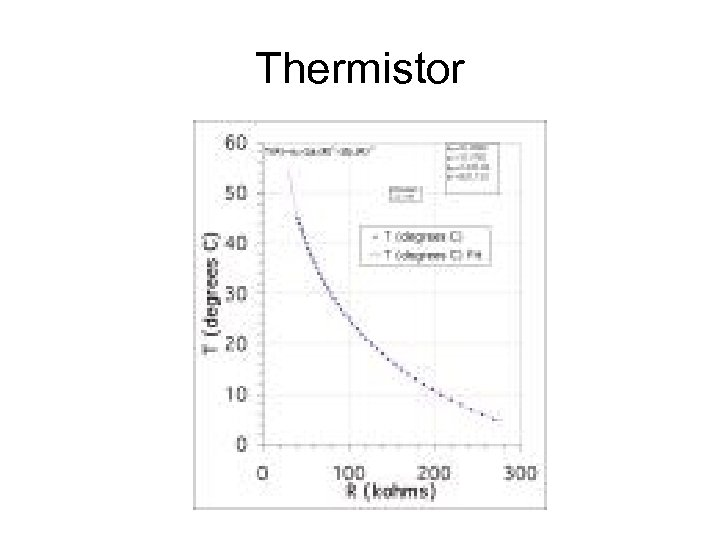Thermistor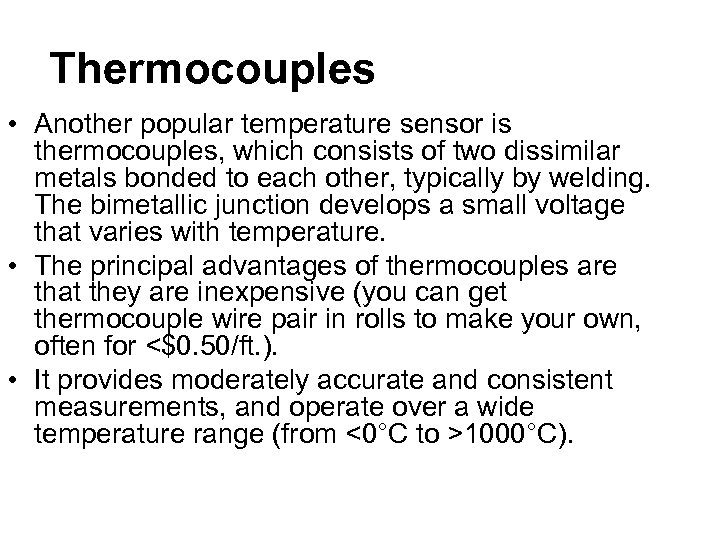Thermocouples • Another popular temperature sensor is thermocouples, which consists of two dissimilar metals bonded to each other, typically by welding. The bimetallic junction develops a small voltage that varies with temperature. • The principal advantages of thermocouples are that they are inexpensive (you can get thermocouple wire pair in rolls to make your own, often for <\$0. 50/ft. ). • It provides moderately accurate and consistent measurements, and operate over a wide temperature range (from <0°C to >1000°C).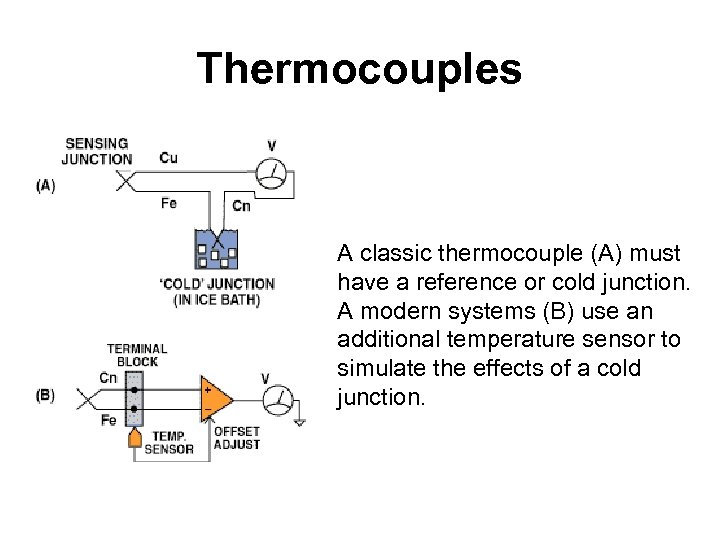Thermocouples A classic thermocouple (A) must have a reference or cold junction. A modern systems (B) use an additional temperature sensor to simulate the effects of a cold junction.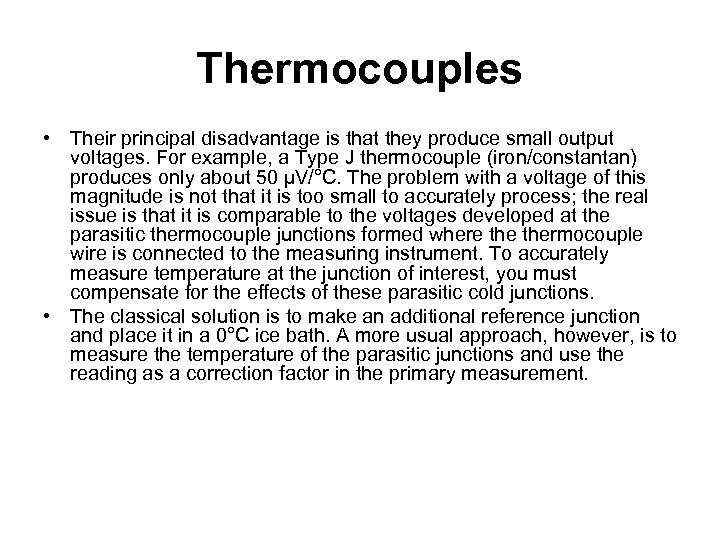Thermocouples • Their principal disadvantage is that they produce small output voltages. For example, a Type J thermocouple (iron/constantan) produces only about 50 µV/°C. The problem with a voltage of this magnitude is not that it is too small to accurately process; the real issue is that it is comparable to the voltages developed at the parasitic thermocouple junctions formed where thermocouple wire is connected to the measuring instrument. To accurately measure temperature at the junction of interest, you must compensate for the effects of these parasitic cold junctions. • The classical solution is to make an additional reference junction and place it in a 0°C ice bath. A more usual approach, however, is to measure the temperature of the parasitic junctions and use the reading as a correction factor in the primary measurement.P-N Junctions • Semiconductor devices can also be used to measure temperature. At a constant current bias, the voltage drop across a silicon P-N diode junction shows roughly a – 2 m. V/ °C temperature coefficient. • Because the P-N junction is the basic building block of diodes, transistors, and ICs, temperature sensing can be incorporated in many devices at low cost. This technique is used in the onboard temperature sensors of microprocessors (e. g. , Intel’s Pentium) and for thermal-shutdown circuits of power-supply chips. • Next shows how a 1 N 914 diode can be used as an inexpensive (<\$0. 05) temperature probe.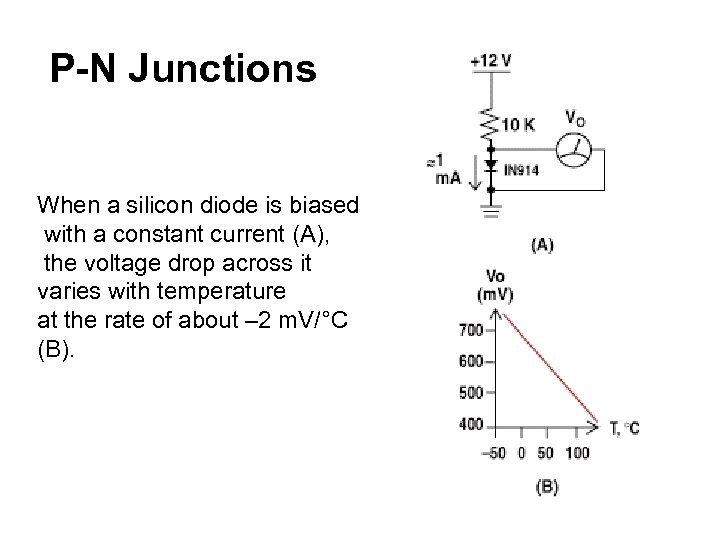P-N Junctions When a silicon diode is biased with a constant current (A), the voltage drop across it varies with temperature at the rate of about – 2 m. V/°C (B).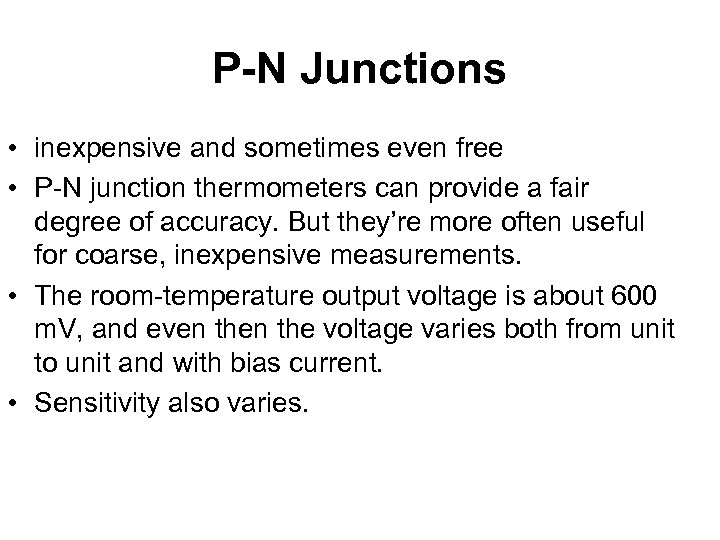P-N Junctions • inexpensive and sometimes even free • P-N junction thermometers can provide a fair degree of accuracy. But they’re more often useful for coarse, inexpensive measurements. • The room-temperature output voltage is about 600 m. V, and even the voltage varies both from unit to unit and with bias current. • Sensitivity also varies.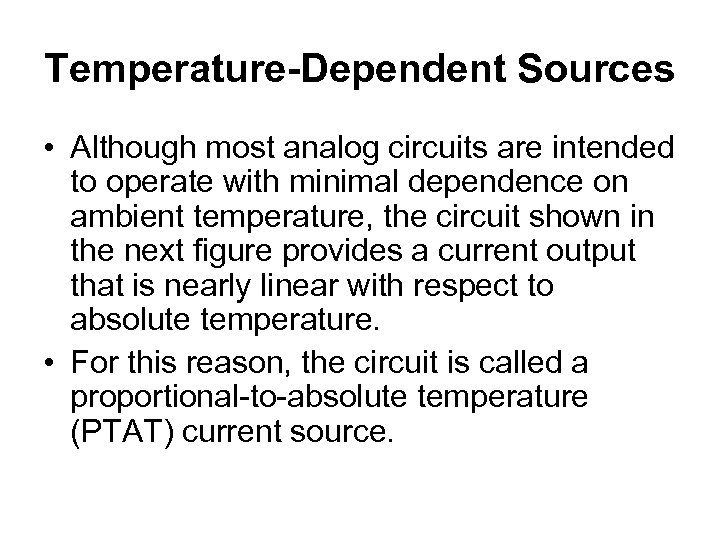Temperature-Dependent Sources • Although most analog circuits are intended to operate with minimal dependence on ambient temperature, the circuit shown in the next figure provides a current output that is nearly linear with respect to absolute temperature. • For this reason, the circuit is called a proportional-to-absolute temperature (PTAT) current source.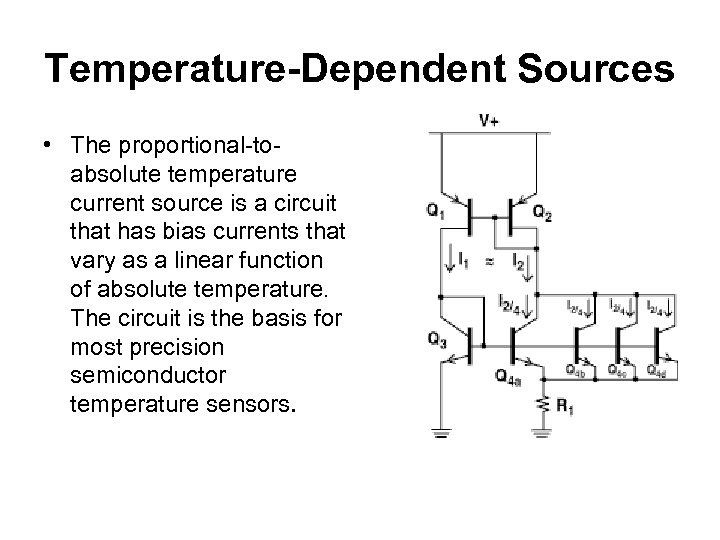Temperature-Dependent Sources • The proportional-toabsolute temperature current source is a circuit that has bias currents that vary as a linear function of absolute temperature. The circuit is the basis for most precision semiconductor temperature sensors.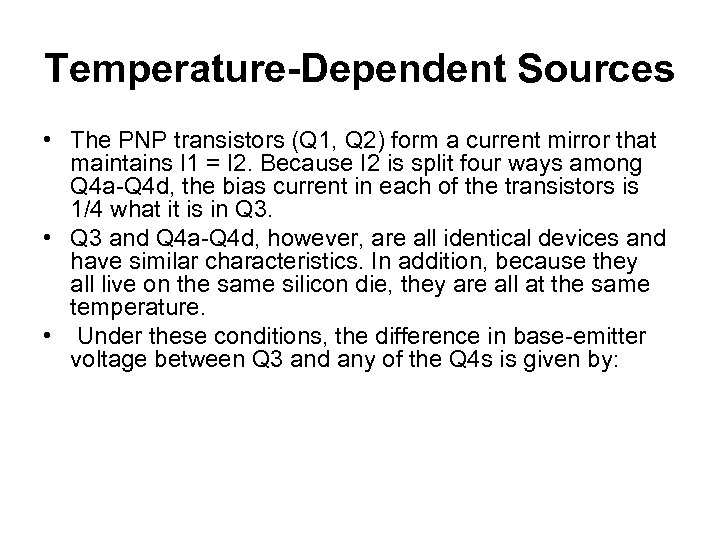Temperature-Dependent Sources • The PNP transistors (Q 1, Q 2) form a current mirror that maintains I 1 = I 2. Because I 2 is split four ways among Q 4 a-Q 4 d, the bias current in each of the transistors is 1/4 what it is in Q 3. • Q 3 and Q 4 a-Q 4 d, however, are all identical devices and have similar characteristics. In addition, because they all live on the same silicon die, they are all at the same temperature. • Under these conditions, the difference in base-emitter voltage between Q 3 and any of the Q 4 s is given by: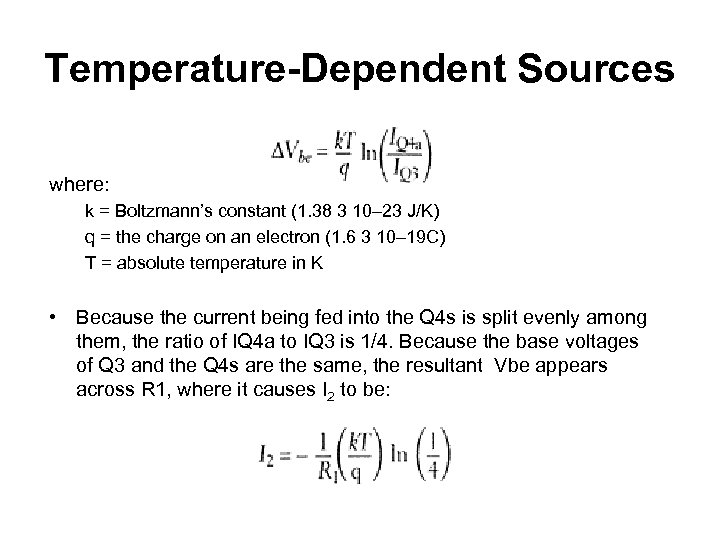Temperature-Dependent Sources where: k = Boltzmann’s constant (1. 38 3 10– 23 J/K) q = the charge on an electron (1. 6 3 10– 19 C) T = absolute temperature in K • Because the current being fed into the Q 4 s is split evenly among them, the ratio of IQ 4 a to IQ 3 is 1/4. Because the base voltages of Q 3 and the Q 4 s are the same, the resultant Vbe appears across R 1, where it causes I 2 to be: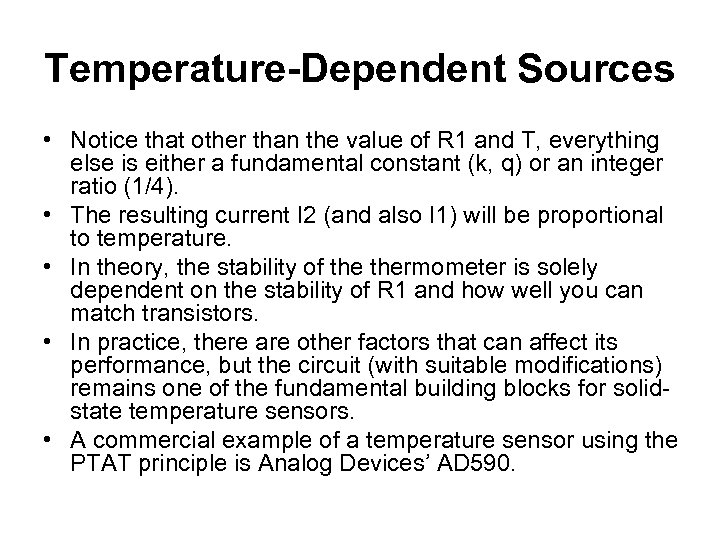Temperature-Dependent Sources • Notice that other than the value of R 1 and T, everything else is either a fundamental constant (k, q) or an integer ratio (1/4). • The resulting current I 2 (and also I 1) will be proportional to temperature. • In theory, the stability of thermometer is solely dependent on the stability of R 1 and how well you can match transistors. • In practice, there are other factors that can affect its performance, but the circuit (with suitable modifications) remains one of the fundamental building blocks for solidstate temperature sensors. • A commercial example of a temperature sensor using the PTAT principle is Analog Devices’ AD 590.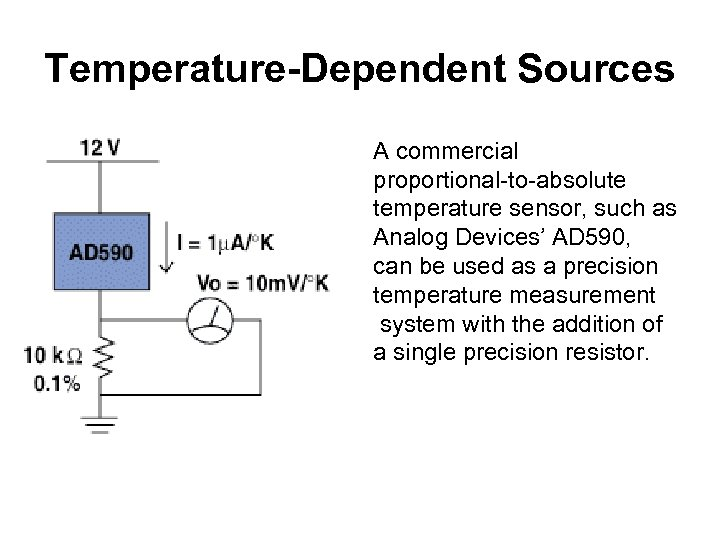Temperature-Dependent Sources A commercial proportional-to-absolute temperature sensor, such as Analog Devices’ AD 590, can be used as a precision temperature measurement system with the addition of a single precision resistor.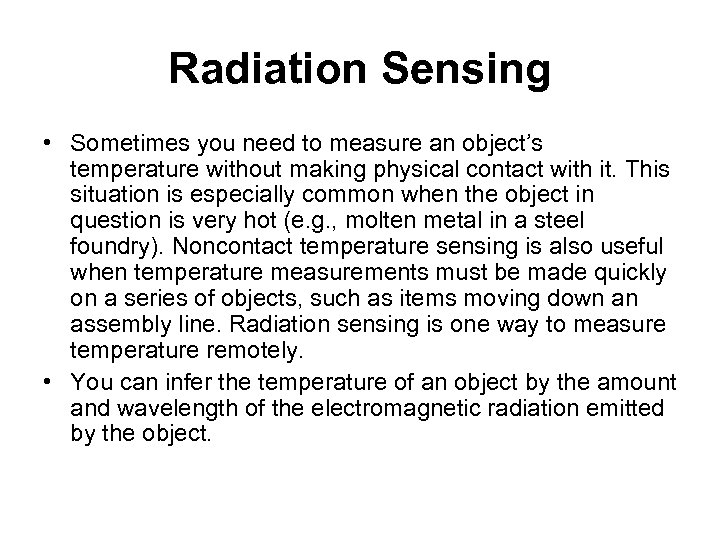Radiation Sensing • Sometimes you need to measure an object’s temperature without making physical contact with it. This situation is especially common when the object in question is very hot (e. g. , molten metal in a steel foundry). Noncontact temperature sensing is also useful when temperature measurements must be made quickly on a series of objects, such as items moving down an assembly line. Radiation sensing is one way to measure temperature remotely. • You can infer the temperature of an object by the amount and wavelength of the electromagnetic radiation emitted by the object.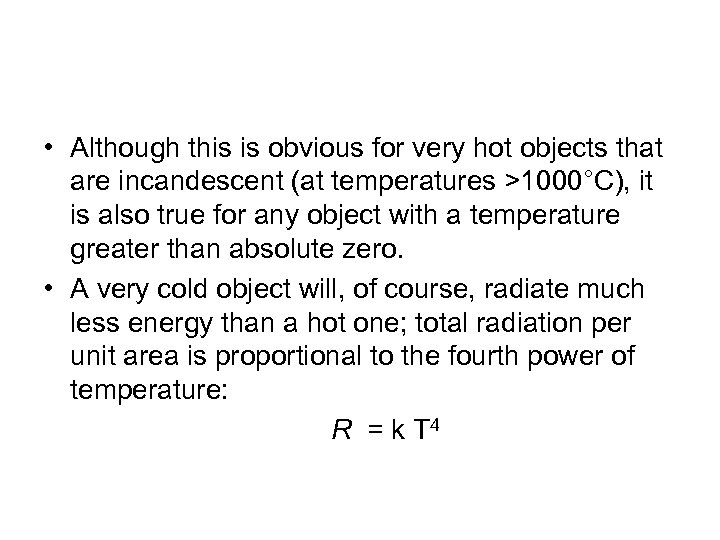• Although this is obvious for very hot objects that are incandescent (at temperatures >1000°C), it is also true for any object with a temperature greater than absolute zero. • A very cold object will, of course, radiate much less energy than a hot one; total radiation per unit area is proportional to the fourth power of temperature: R = k T 4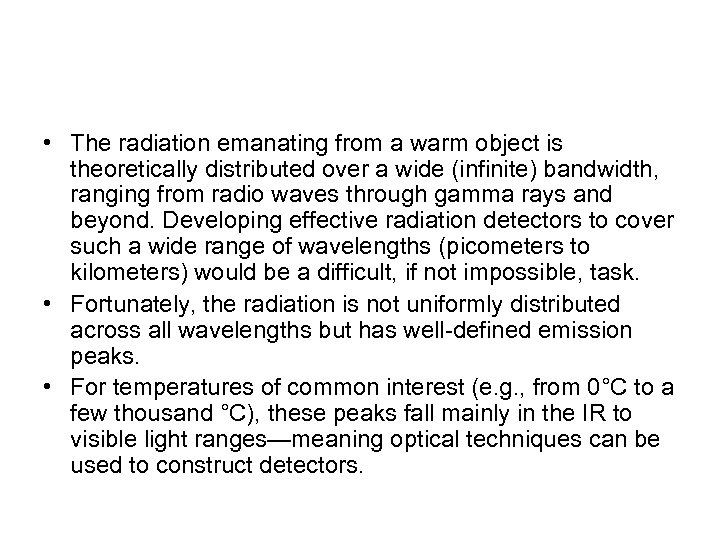• The radiation emanating from a warm object is theoretically distributed over a wide (infinite) bandwidth, ranging from radio waves through gamma rays and beyond. Developing effective radiation detectors to cover such a wide range of wavelengths (picometers to kilometers) would be a difficult, if not impossible, task. • Fortunately, the radiation is not uniformly distributed across all wavelengths but has well-defined emission peaks. • For temperatures of common interest (e. g. , from 0°C to a few thousand °C), these peaks fall mainly in the IR to visible light ranges—meaning optical techniques can be used to construct detectors.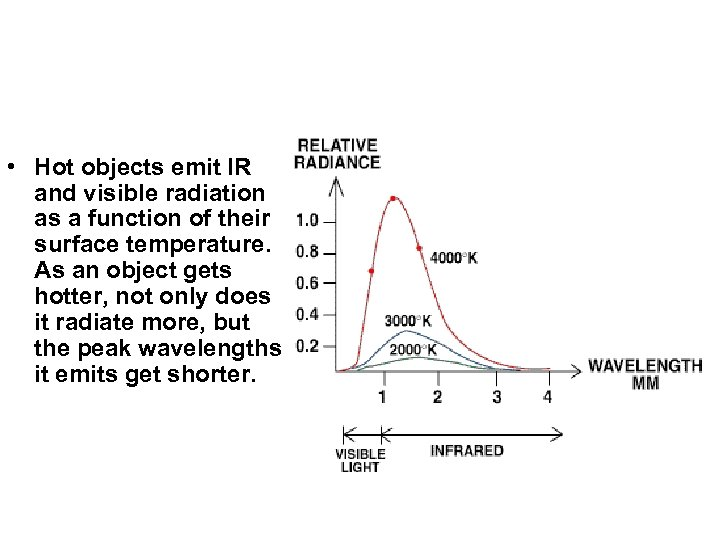• Hot objects emit IR and visible radiation as a function of their surface temperature. As an object gets hotter, not only does it radiate more, but the peak wavelengths it emits get shorter.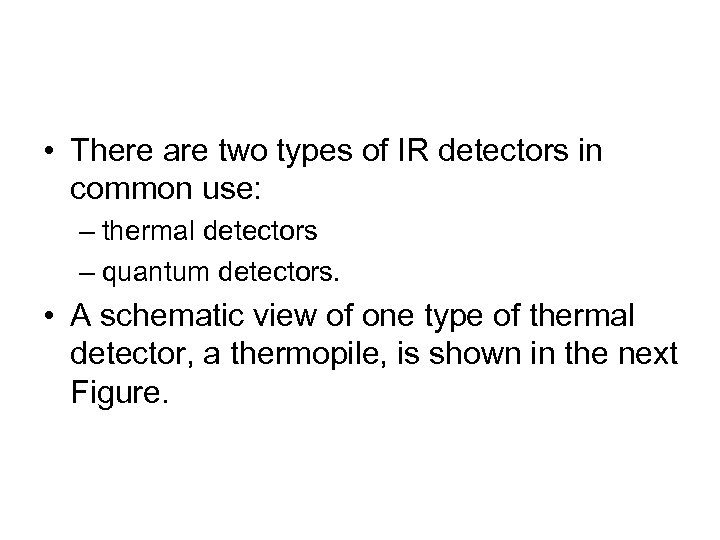• There are two types of IR detectors in common use: – thermal detectors – quantum detectors. • A schematic view of one type of thermal detector, a thermopile, is shown in the next Figure.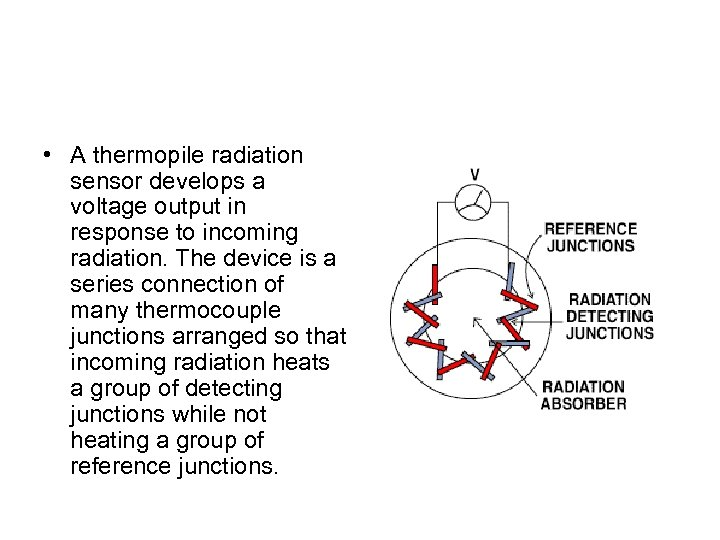• A thermopile radiation sensor develops a voltage output in response to incoming radiation. The device is a series connection of many thermocouple junctions arranged so that incoming radiation heats a group of detecting junctions while not heating a group of reference junctions.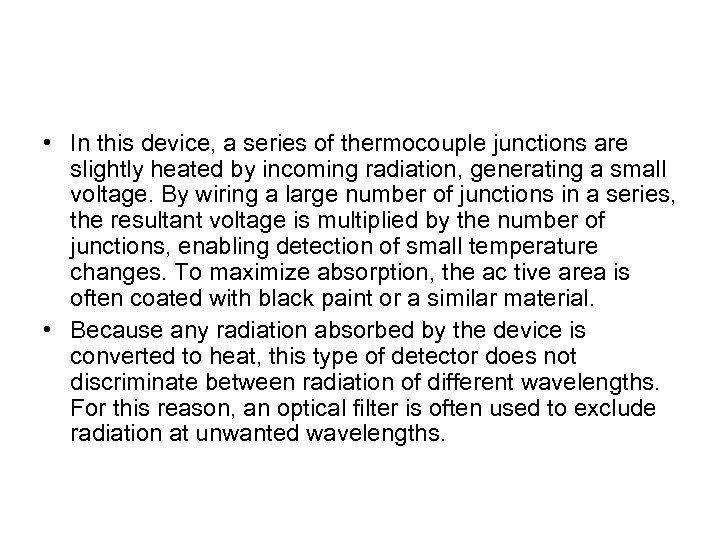• In this device, a series of thermocouple junctions are slightly heated by incoming radiation, generating a small voltage. By wiring a large number of junctions in a series, the resultant voltage is multiplied by the number of junctions, enabling detection of small temperature changes. To maximize absorption, the ac tive area is often coated with black paint or a similar material. • Because any radiation absorbed by the device is converted to heat, this type of detector does not discriminate between radiation of different wavelengths. For this reason, an optical filter is often used to exclude radiation at unwanted wavelengths.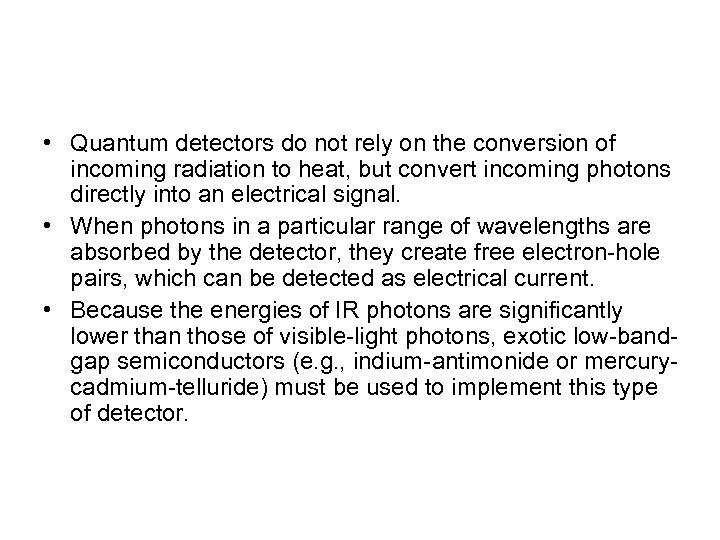• Quantum detectors do not rely on the conversion of incoming radiation to heat, but convert incoming photons directly into an electrical signal. • When photons in a particular range of wavelengths are absorbed by the detector, they create free electron-hole pairs, which can be detected as electrical current. • Because the energies of IR photons are significantly lower than those of visible-light photons, exotic low-bandgap semiconductors (e. g. , indium-antimonide or mercurycadmium-telluride) must be used to implement this type of detector.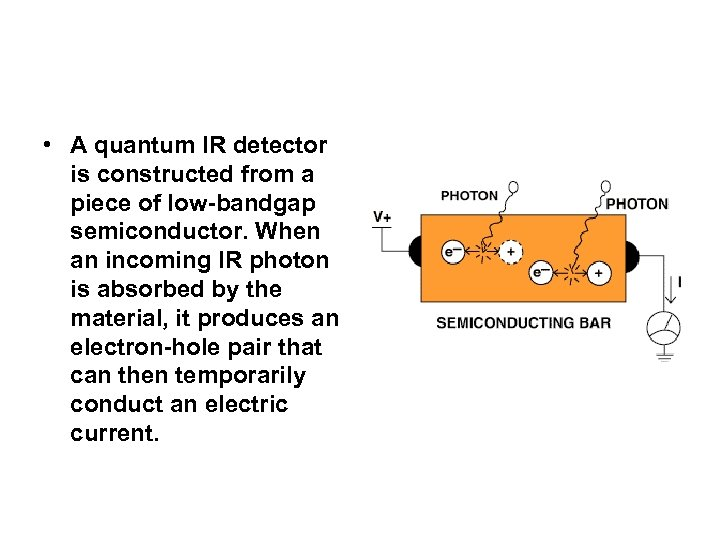• A quantum IR detector is constructed from a piece of low-bandgap semiconductor. When an incoming IR photon is absorbed by the material, it produces an electron-hole pair that can then temporarily conduct an electric current.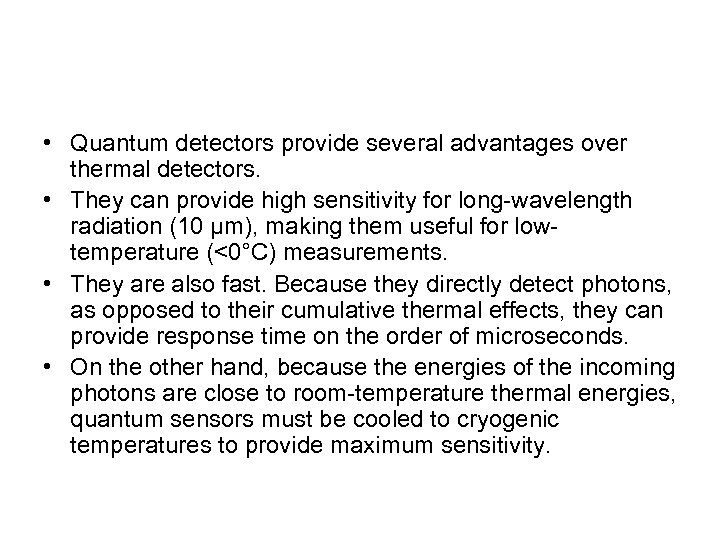• Quantum detectors provide several advantages over thermal detectors. • They can provide high sensitivity for long-wavelength radiation (10 µm), making them useful for lowtemperature (<0°C) measurements. • They are also fast. Because they directly detect photons, as opposed to their cumulative thermal effects, they can provide response time on the order of microseconds. • On the other hand, because the energies of the incoming photons are close to room-temperature thermal energies, quantum sensors must be cooled to cryogenic temperatures to provide maximum sensitivity.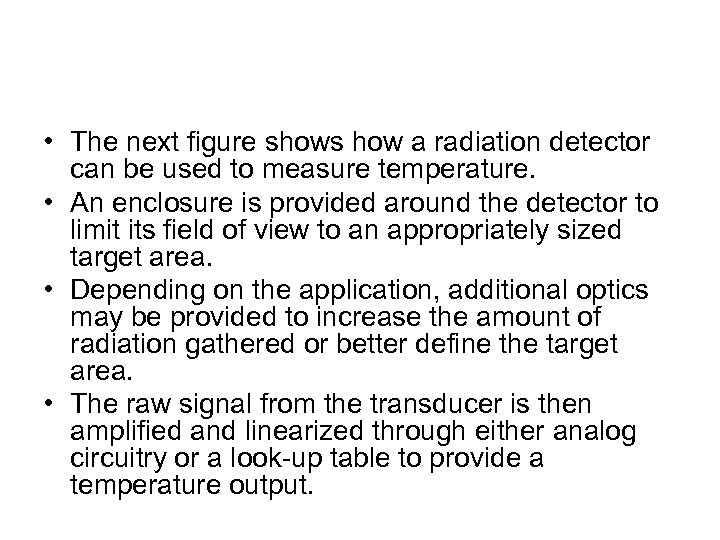• The next figure shows how a radiation detector can be used to measure temperature. • An enclosure is provided around the detector to limit its field of view to an appropriately sized target area. • Depending on the application, additional optics may be provided to increase the amount of radiation gathered or better define the target area. • The raw signal from the transducer is then amplified and linearized through either analog circuitry or a look-up table to provide a temperature output.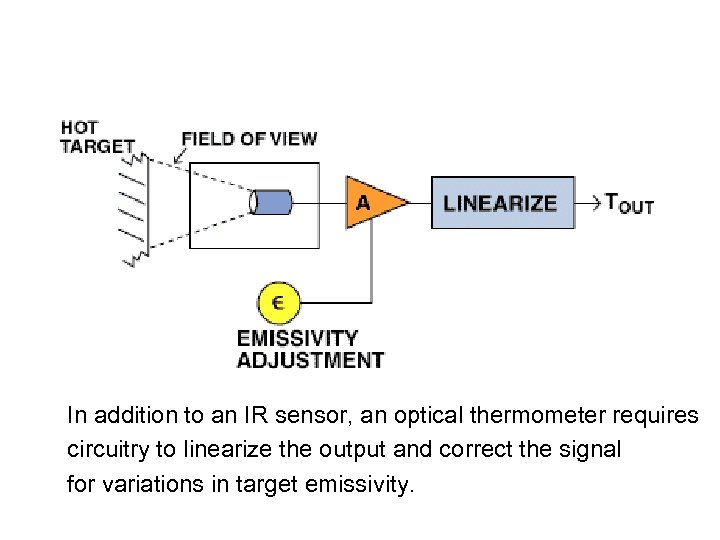In addition to an IR sensor, an optical thermometer requires circuitry to linearize the output and correct the signal for variations in target emissivity.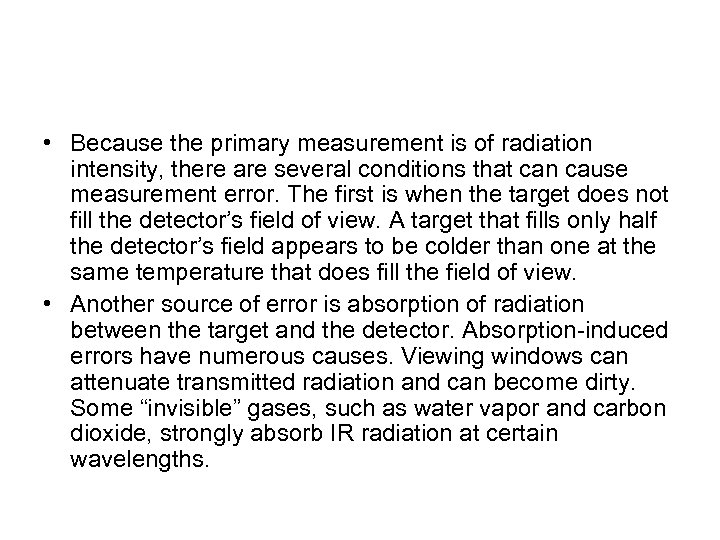• Because the primary measurement is of radiation intensity, there are several conditions that can cause measurement error. The first is when the target does not fill the detector’s field of view. A target that fills only half the detector’s field appears to be colder than one at the same temperature that does fill the field of view. • Another source of error is absorption of radiation between the target and the detector. Absorption-induced errors have numerous causes. Viewing windows can attenuate transmitted radiation and can become dirty. Some “invisible” gases, such as water vapor and carbon dioxide, strongly absorb IR radiation at certain wavelengths.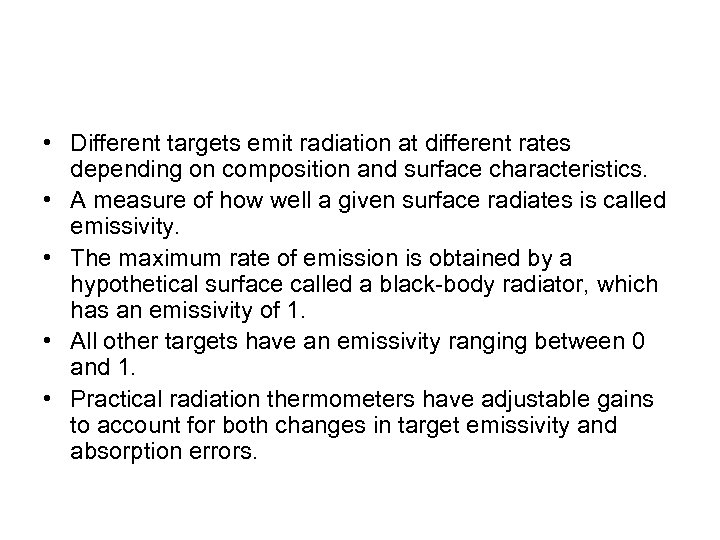• Different targets emit radiation at different rates depending on composition and surface characteristics. • A measure of how well a given surface radiates is called emissivity. • The maximum rate of emission is obtained by a hypothetical surface called a black-body radiator, which has an emissivity of 1. • All other targets have an emissivity ranging between 0 and 1. • Practical radiation thermometers have adjustable gains to account for both changes in target emissivity and absorption errors.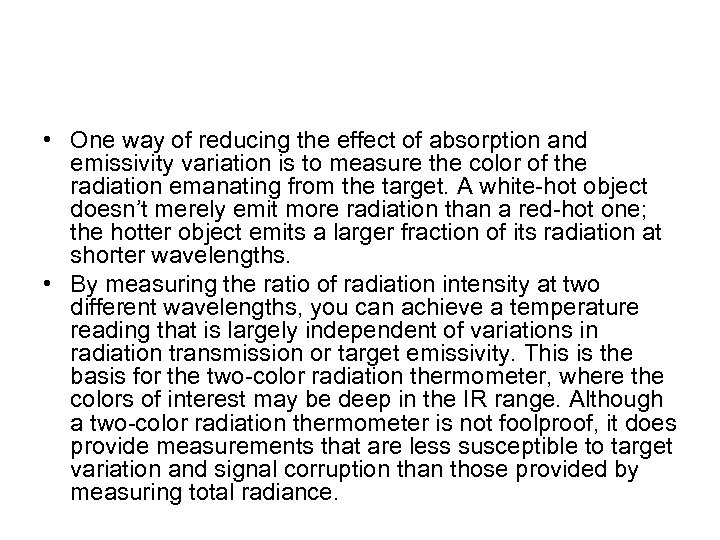• One way of reducing the effect of absorption and emissivity variation is to measure the color of the radiation emanating from the target. A white-hot object doesn’t merely emit more radiation than a red-hot one; the hotter object emits a larger fraction of its radiation at shorter wavelengths. • By measuring the ratio of radiation intensity at two different wavelengths, you can achieve a temperature reading that is largely independent of variations in radiation transmission or target emissivity. This is the basis for the two-color radiation thermometer, where the colors of interest may be deep in the IR range. Although a two-color radiation thermometer is not foolproof, it does provide measurements that are less susceptible to target variation and signal corruption than those provided by measuring total radiance.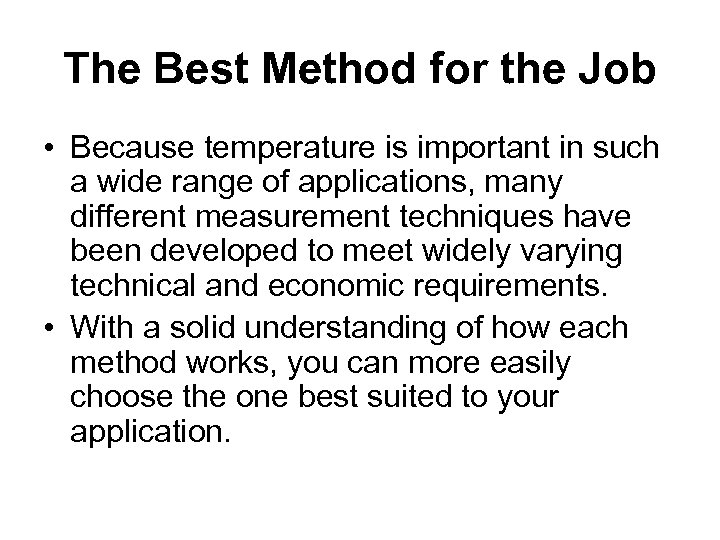The Best Method for the Job • Because temperature is important in such a wide range of applications, many different measurement techniques have been developed to meet widely varying technical and economic requirements. • With a solid understanding of how each method works, you can more easily choose the one best suited to your application.